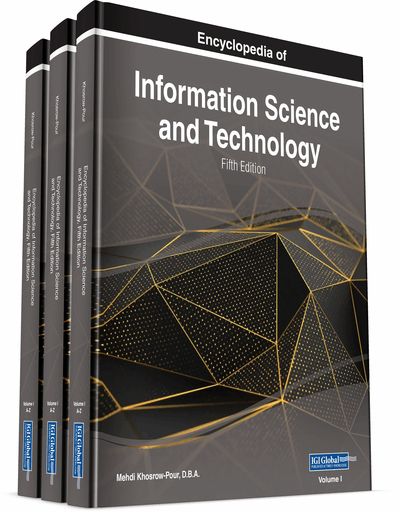# Big Data Mining Algorithms

DOI: 10.4018/978-1-7998-3479-3.ch052
OnDemand:
(Individual Chapters)
Available
\$29.50
No Current Special Offers

## Abstract

Big data mining involves knowledge discovery from these large data sets. The purpose of this chapter is to provide an analysis of different machine learning algorithms available for performing big data analytics. The machine learning algorithms are categorized in three key categories, namely, supervised, unsupervised, and semi-supervised machine learning algorithm. The supervised learning algorithms are trained with a complete set of data, and thus, the supervised learning algorithms are used to predict/forecast. Example algorithms include logistic regression and the back propagation neural network. The unsupervised learning algorithms starts learning from scratch, and therefore, the unsupervised learning algorithms are used for clustering. Example algorithms include: the Apriori algorithm and K-Means. The semi-supervised learning combines both supervised and unsupervised learning algorithms. The semi-supervised algorithms are trained, and the algorithms also include non-trained learning.
Chapter Preview
Top

## Focus Of The Article

Machine learning is a field of computer science that try to enable computers with the ability to learn without explicitly programming them. The purpose of this chapter is to provide an analysis of different Machine learning algorithms available for performing big data analytics. The ML algorithms are categorized in three key categories, namely, supervised, unsupervised and semi-supervised ML algorithm. The Big Data Analytics or Big Data Security analytics fully depends on Data Mining, where the Machine Learning techniques are subset of Data Mining (Ripon Patgiri, 2018).

### Supervised Learning Algorithms

The supervised learning algorithms are trained with a complete set of data and thus, the supervised learning algorithms are used to predict/forecast.

Example problems are classification and regression. Example algorithms include: Logistic Regression and the Back Propagation Neural Network.

## Key Terms in this Chapter

Unsupervised Learning Algorithms: The unsupervised learning algorithms starts learning from scratch, and therefore, the unsupervised learning algorithms are used for clustering.

Ensemble Algorithms: It combines multiple learning algorithms to obtain better predictive performance.

Deep Learning Algorithms: It uses unsupervised learning to set each level of hierarchy of features using features discovered at previous level.

Regression Algorithm: It predict output values using input features of the data provided to the system.

Association Rule Learning Algorithms: It is used to discover relationship between data points.

Supervised Learning Algorithms: The supervised learning algorithms are trained with a complete set of data and thus, the supervised learning algorithms are used to predict/forecast.

Data Mining: Data mining is widely used in fields such as science, engineering, medicine, and business.

Semi-Supervised Learning Algorithms: The semi-supervised learning combines both supervised and unsupervised learning algorithms.

Decision Tree Algorithm: It solves the problem using tree representation.

## Complete Chapter List

Search this Book:
Reset